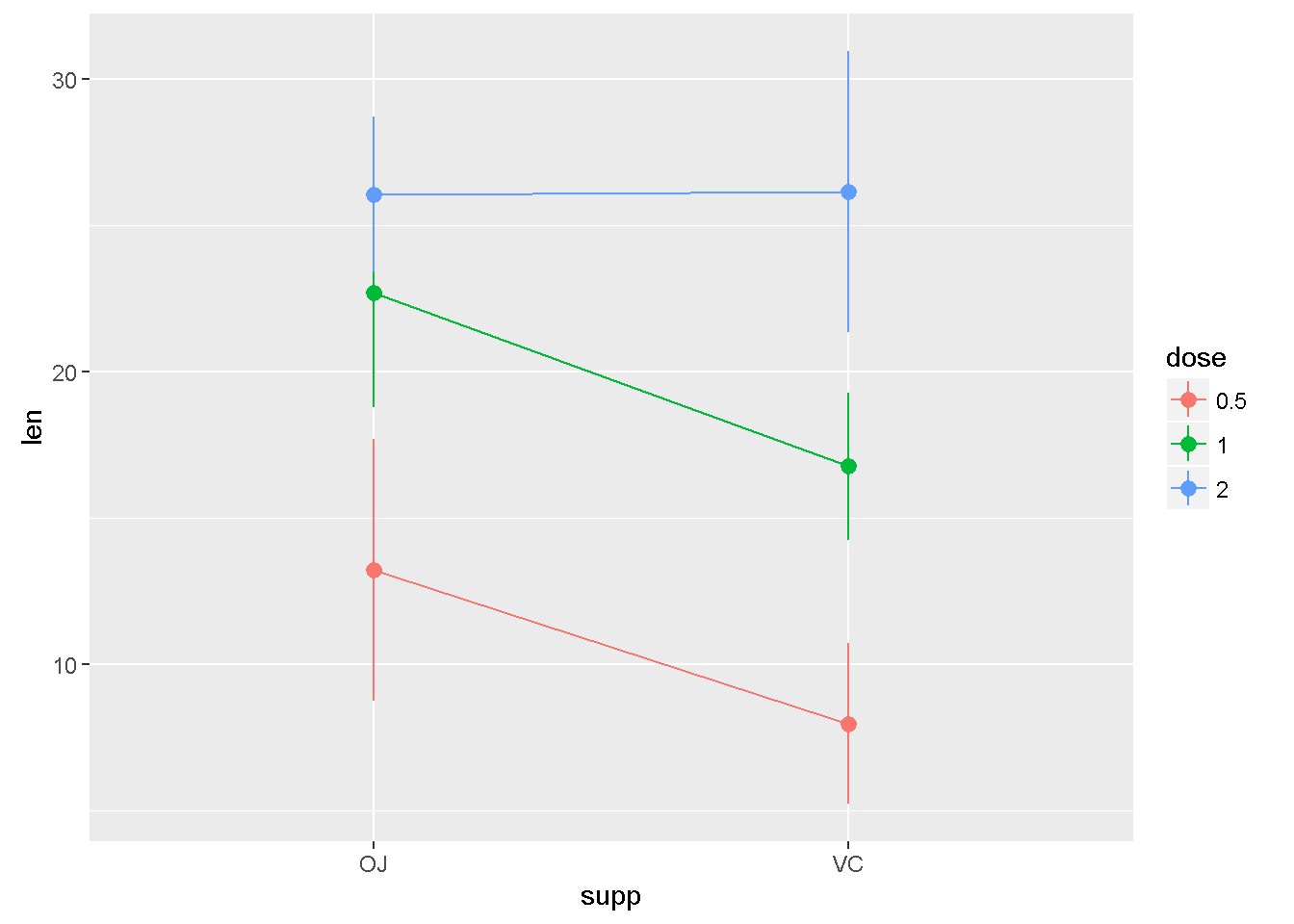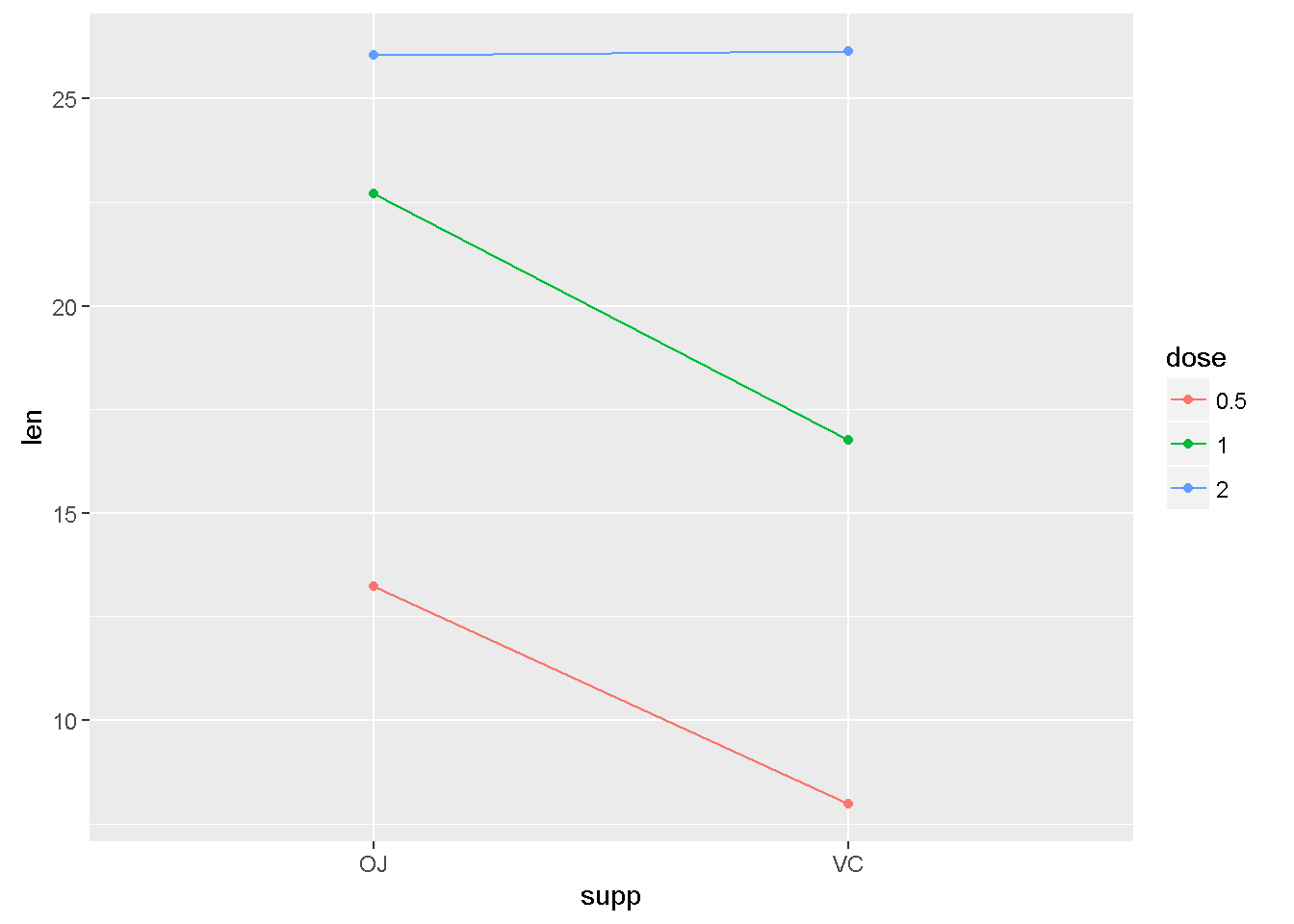Some grouped data

data(ToothGrowth)
ToothGrowth$dose <- factor(ToothGrowth$dose)

w/ stat_summary(…geom = “pointrange”)

library(ggplot2)
ggplot(ToothGrowth,
aes(y = len, x = supp, colour = dose, group = dose)) +
stat_summary(fun.y = mean,
fun.ymin = function(x) mean(x) - sd(x),
fun.ymax = function(x) mean(x) + sd(x),
geom = "pointrange") +
stat_summary(fun.y = mean,
geom = "line")w/ geom = “point”

ggplot(ToothGrowth, aes(y = len, x = supp, colour = dose, group = dose)) +
stat_summary(fun.y = mean,
geom = "point") +
stat_summary(fun.y = mean,
geom = "line")